# Formula Forensics No. 031 – Production Scheduling using Excel

Share

Recently, Bluetaurean asked in the Chandoo.org Forums about ways to allocate work durations for various product lines across 24 hour days to create a daily schedule.

Both formula-based and VBA-based solutions were offered.

Today at formula Forensics we will take a look at the formula-based approach.

## Set the Scene

Since one might encounter a similar need in a variety of contexts (manufacturing, engineering, project planning, etc.), we will look at a more general problem of allocating a set of tasks and corresponding durations to one or more days, as shown in the following diagram.

We will create two output views:

• One that is a flat list that can then be manipulated further using Excel’s Pivot table feature, and
• Another view that mimics a pivot-table (and is similar to a typical project Gantt view, but with actual values listed instead of a bar chart).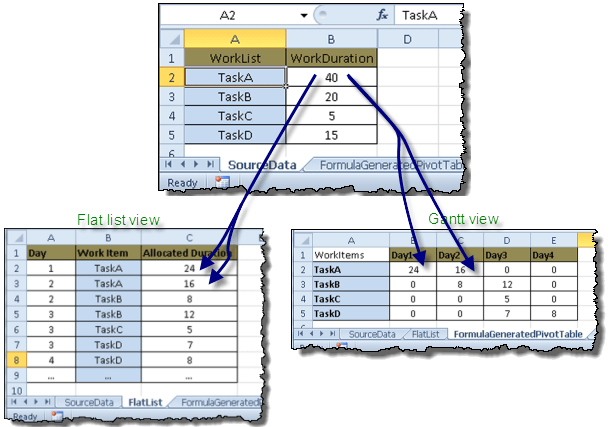## Problem Specifics

• We have a list of tasks and their durations.
• We need to distribute the tasks to different days, without exceeding the maximum available duration in a given day.
• When the hours in a day are “used up”, we need to allocate the remaining task duration to the next day, and so on.
• On the other hand, if a given task does not use up all of the hours in a given day, we will need to assign more than one task for that day, provided the combined durations do not exceed the available hours for that day.
• In other words, we will need to split a task across one or more days, or combine one or more tasks into a single day, as needed, to maximize the work performed in a given day.

## Developing the Approach

Before we tackle this problem in Excel, let us review how we might do this manually. Like most things, we might use the following three step process:

1. Take the first task and assign its duration to Day 1. If the task’s duration exceeds the maximum hours available in a day, allocate the portion of the duration that does not fit into Day 1 into Day 2.
2. Take the second task, and see whether it can fit into an existing day, or whether it needs to be distributed to multiple days
3. Etc. (OK… so that three-step process was a stretch!)

Statistics show that most people think in terms of IF-THEN-ELSE statements. So here it is…

For a given Day, and for a given Task,
If [Hours Not Allocated For that Task] > [Hours Available for that Day] Then
Set Duration for that Day as [Hours Available for that Day]
Else
Set Duration for that Day as [Hours Not Allocated for that Task]
End
Continue the above evaluation until all tasks have been allocated to days.

Of course, the above IF() logic can be condensed as follows:

MIN( [Hours Not Allocated For that Task][Hours Available for that Day] )

## Putting it All Together: Output Option 1: Gantt-like View

Let us employ the above approach to create the Gantt-like view.

To make our approach more generic, we will use an Excel Name called “MaxHrsPerDay” to indicate the maximum available hours in a given day. (In the sample worksheet, it has been set to 24 hours.)

Our source data is setup as shown in the diagram below:

• Tasks are in the range A2:A5
• Durations are in the range B2:B5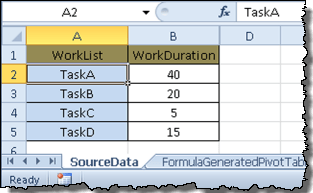We will create the output in a separate worksheet, in the range A1:E5 as shown below: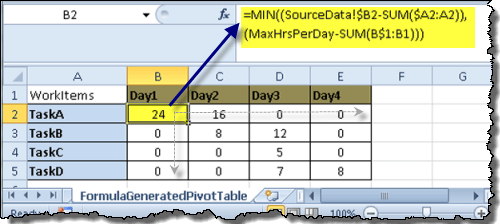Put the following formula into cell A2 and copy down to A5:

=SourceData!\$A2

(This formula is merely referencing the values from the SourceData sheet. The sample workbook also includes an approach to make this reference more location independent.)

Put the following formula in cell B2, and copy it down and right:

=MIN((SourceData!\$B2-SUM(\$A2:A2)), (MaxHrsPerDay-SUM(B\$1:B1)))

Setup the header row (B1:E1) as desired. (I have used text values for the header. You could also calculate the header text using formulas. Since that is straightforward, I will leave that as an exercise for the reader.)

Now let us look at what the formula in cell B2 is doing:

• SUM(\$A2:A2) is calculating the sum of the allocated durations for TaskA. (Please note the use of absolute and relative references. The formula is anchored on column A, but the starting row, ending row and ending column are free to expand.) SUM(\$A2:A2) returns zero since SUM() ignores text values.

– If you look at cell C2, the reference changes to SUM(\$A2:B2).
– In cell B3, the reference changes to SUM(\$A3:A3). You get the idea

• (SourceData!\$B2-SUM(\$A2:A2)) calculates the difference between the duration for TaskA (40 in the example) and the hours allocated as of that point (0), to return 40-0=40.
• SUM(B\$1:B1) is calculating the sum of the allocated hours for Day1. (Again, we are using a combination of absolute and relative references to keep the calculation anchored on column B.) In this case, the value is zero, since this is the first allocation for Day1.
• (MaxHrsPerDay-SUM(B\$1:B1)) calculates the hours remaining (i.e. available) for Day1. Since this is for cell B2, the calculation returns 24 – 0 = 24.

That is it!

We put those absolute and relative references to good use!

This approach was easy because all we had to do was calculate the duration for a given task for a given day.

On the other hand, if we had to figure out what the Task was, or which Day it was, the calculation gets a little more involved. Since this is “formula forensics”, we would not have it any other way! 🙂

## Putting it All Together: Output Option 2: A Sequential List of Tasks and Durations for Each Day (i.e. a Flat List)

As before, we will use the Excel Name “MaxHrsPerDay” to refer to the maximum hours in a Day.

As shown in the following diagram, we will turn the source data into a flat list of Days, Tasks and Durations:Unlike with VBA, since a formula cannot choose which row and column to write its output, we have to set the formula in every cell where we suspect there might be a value.

In the above sample diagram, we copy the formulas from row 2 to row 9. However, row 9 shows “…” indicating that the list was completed by row 8.

Let us look at how to determine the value for Day, Task and Allocated Duration.

For ease of description, I have created the following Excel Names:

WorkList: =A2:A5 in the source data.

WorkDuration: =B2:B5 in the source data

While creating the Gantt-like view earlier, we were able to take advantage of the static “Day” and “Task” values to determine the Remaining Duration, Available Duration, etc. Since we now have to determine all three values (Day, Task, Allocated Duration), we will need some “helper” data.

We will add a column alongside the source data that shows the cumulative duration (for reasons that will become clear shortly), as shown in the following diagram:Cumulative Duration is calculated as the sum of all durations up to a given row.

• For example, in cell C2, the Cumulative Duration is 40.
• In cell C3, the Cumulative Duration is 40+20=60
• And so on.

For ease of referencing, we will use an Excel Name called CumulativeDuration =C2:C5.

### The circular logic problem

In order to determine the durations already allocated for a given day, we will need to know which Day it is.

We also need to know which Task we are trying to calculate the duration for.

So… do we calculate the Day or the Task or the Duration first?!! As you can imagine, that will soon land us in some circular logic.

• In column C of the output (on worksheet FlatList), the sum of allocated durations adds up to the total duration for all tasks. (No surprise here!)
• If every task had duration equal to the MaxHrsPerDay, you would have the same duration value for all days. (Not surprising, but interesting!)
• In other words, you could think of the Allocated Duration column as the total duration for all tasks, allocated MaxHrsPerDay at a time.
• Now we need a way to iterate through the duration values one at a time and account for the durations already processed. In other words, each value needs to contain all of the previous values. Welcome to an array of the cumulative durations!
• For example, in the cumulative array “{40;60;65;80}”, the value 60 already includes the previous value 40 in it. This allows us to subtract all durations allocated up to a given row, to get the duration value that is remaining to be allocated.
• Since Excel is good with numbers, we will base the calculation for AllocatedDuration and Tasks on the Duration values.
• By calculating the two values separately, we avoid the circular logic.

Let’s now look at the formulas for Day, WorkItem and AllocatedDuration.

It would be easier if we looked at the formulas in reverse order, starting with AllocatedDuration, then WorkItem, and finally Day.

### Formula for “AllocatedDuration”

Enter the following formula into cell C2, ending with Ctrl+Shift+Enter, as shown in the following diagram:

=IF(SUM(C\$1:C1)>=SUMPRODUCT(WorkDuration), “…”,MIN(INDEX(WorkDuration, MATCH(TRUE, CumulativeDuration-SUM(C\$1:C1) > 0, 0)) – SUMIFS(C\$1:C1, B\$1:B1,B2), MaxHrsPerDay-SUMPRODUCT((A\$1:A1=A2)* IF(ISNUMBER(C\$1:C1), C\$1:C1, 0)))) Ctrl+Shift+Enter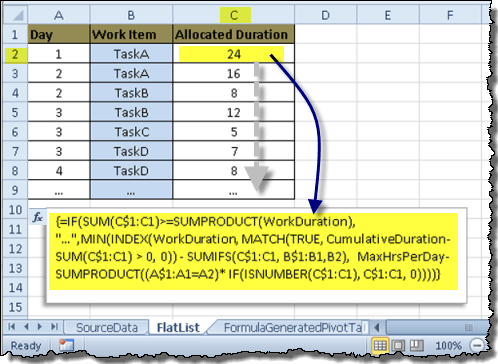Let us look at the formula closely (using the formula in row 2):

• SUMPRODUCT((A\$1:A1=A2)* IF(ISNUMBER(C\$1:C1), C\$1:C1, 0)) -> This calculates the sum of all allocated durations up to the previous row, where the Day = current row’s day. Please note the use of absolute and relative references. They allow us to expand the range as we go down the rows, while remaining anchored to the first row.

– Since this is the first data row, C\$1:C1 returns “Allocated Duration” and the ISNUMBER() function returns FALSE, and consequently, the IF() function returns 0.
– A\$1:A1 returns “Day”, and the test A\$1:A1=A2 returns FALSE. Please note that in this case, it does not matter whether A2 has a value in it, whether it has the value 1, etc.
– SUMPRODUCT() provides the result of FALSE * 0 = 0

• MaxHrsPerDaySUMPRODUCT((A\$1:A1=A2)* IF(ISNUMBER(C\$1:C1), C\$1:C1, 0)) -> This calculates the difference between maximum duration available for a day and the sum of durations allocated for the current day. In other words, it calculates the available duration for the current row’s day.

– In this example, the calculation results in MaxHrsPerDay (24 in our example) – 0 = 24

• SUMIFS(C\$1:C1, B\$1:B1,B2) -> This calculates the sum of all allocated durations for the current row’s task. Since B\$1:B1 is the text value “Work Item”, the SUMIFS() returns 0. Again, it does not matter if B2 is blank or has a value like “TaskA”, since Excel correctly evaluates the condition whether B\$1:B1 equals B2.
• SUM(C\$1:C1) -> This calculates the sum of all allocated durations up to the previous row.
• CumulativeDurationSUM(C\$1:C1) -> CumulativeDuration evaluates to {40;60;65;80}. SUM(C\$1:C1) evaluates to zero. As such, the expression evaluates to {40;60;65;80} – 0, or {40;60;65;80}.

– If we look at the calculation for this expression in cell C3 (the expression would be “CumulativeDuration—SUM(C\$1:C2)”), we would get the result of {40;60;65;80} – (0+24) = {16;36;41;56}. (As you know, subtracting a scalar value from an array results in an array with each value reduced by the scalar value.)

– If we look at the calculation for this expression in cell C4 (the expression would be “CumulativeDuration—SUM(C\$1:C3)”) , we would get the result of {40;60;65;80} – (0+24+16) = {0;20;25;40}

– As you can see, each successive calculation reduces the CumulativeDuration array by the amount of hours already allocated. By reducing the CumulativeDuration array in this fashion, we ensure that we do not “double count” a duration.

– If a value in the array evaluates to zero, it means the corresponding duration has been fully allocated. (In cell C3, the first value in the array is zero, indicating that the original 40 hours has been fully allocated.) We will put this knowledge to good use in the next expression.

• MATCH(TRUE, CumulativeDuration—SUM(C\$1:C1) > 0, 0) -> The expression CumulativeDuration—SUM(C\$1:C1) > 0 evaluates to ={TRUE;TRUE;TRUE;TRUE} because all values are greater than zero. By performing a MATCH() for TRUE, we are able to find the first location in the array that has a non-zero value.

– If we look at the result of this expression in cell C3, we get {16;36;41;56} > 0 = {TRUE;TRUE;TRUE;TRUE}

– If we look at the result of this expression in cell C4, we get {0;20;25;40} > 0 = {FALSE;TRUE;TRUE;TRUE}

– As you recall, the zero values (or FALSE) correspond to the durations that have been fully allocated, whereas, the non-zero values (or TRUE) correspond to the durations that have NOT been fully allocated.

– It is helpful to note that MATCH() returns the LOCATION of what it finds. As such, the returned location is that of the first duration value that has not been fully allocated! Since the CumulativeDuration array is the same size as the WorkDuration array, we will be able to put this returned location value to good use in the next expression.

• INDEX(WorkDuration, MATCH(TRUE, CumulativeDuration — SUM(C\$1:C1) > 0, 0)) -> By using the location value (of the first duration value that has not been fully allocated), we find the corresponding original duration value from the WorkDuration array.

– As we saw earlier, the expression “CumulativeDiration – SUM(C\$1:C1)” reduces the CumulativeDuration by the duration values allocated to that point. However, the resulting array could have partial duration values as well. By referencing the corresponding duration value from the WorkDuration array, we ensure that we retrieve the original (full) duration value that was to be allocated.

• MIN(…) -> This expression calculates the value of MIN([Hours Not Allocated For that Task], [Hours Available for that Day])

– [Hours Not Allocated For that Task] is returned by INDEX(WorkDuration, MATCH(TRUE, CumulativeDuration—SUM(C\$1:C1) > 0, 0)) – SUMIFS(C\$1:C1, B\$1:B1,B2)

– [Hours Available for that Day] is returned by second half of the MIN() expression: MaxHrsPerDay—SUMPRODUCT((A\$1:A1=A2)* IF(ISNUMBER(C\$1:C1), C\$1:C1, 0)).

– So, we essentially got back to the logic we started from, which is the same logic we used for creating the Gantt-like view as well.

• The remaining portion of the formula (the IF() check) determines if all of the hours have been allocated. If all hours have been allocated, it returns “…”.

SUMPRODUCT(WorkDuration) -> This expression calculates the total of all work duration values. In cell C2, it evaluates to SUMPRODUCT({40;20;5;15}) = 80

SUM(C\$1:C1)>=SUMPRODUCT(WorkDuration) -> Determines if the sum of durations allocated up to that point is greater than the total for all durations. (Since this is part of an array formula, you could also use the SUM function in place of SUMPRODUCT. But I am partial to the SUMPRODUCT function!! So, unless you are in a competition where the winner is determined by the shortest formula, feel free to use either one!

### Formula for “WorkItem”

Enter the following formula into cell B2, ending with Ctrl+Shift+Enter, as shown in the following diagram.

=IF(SUM(C\$1:C1)>=SUMPRODUCT(WorkDuration), “…”,INDEX(WorkList, MATCH(TRUE, (CumulativeDuration-SUM(C\$1:C1)) > 0, 0))) Ctrl+Shift+EnterYou are already familiar with most of the formula components since you saw them in the formula for AllocatedDuration. The only difference is that in this formula, we are returning a value from WorkList. (i.e. we locate the position of the first non-zero duration in CumulativeDuration array, and since that array is the same size as the WorkList array, we are able to find the first Task that has not been fully allocated.)

### Formula for “Day”

Enter the following formula into cell A2, ending with Ctrl+Shift+Enter, as shown in the following diagram:

=IF(SUM(C\$1:C1)>=SUMPRODUCT(WorkDuration), “…”, MAX( N(A1) + (SUMIFS(C\$1:C1, A\$1:A1, A1)>=MaxHrsPerDay), 1)) Ctrl+Shift+Enter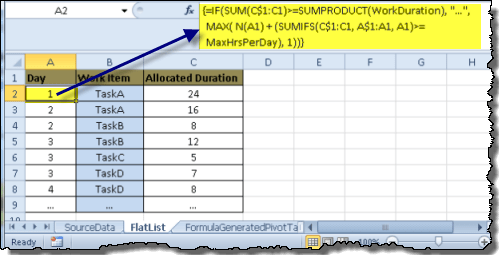Let us look at the formula in detail (using the formula in row 2):

• SUMIFS(C\$1:C1, A\$1:A1, A1) -> This expression calculates the sum of all durations (in column C) where the Days (in column A) equal the previous day.

– In cell A2, this expression evaluates to “SUMIFS(“Allocated Duration”, “Day”, “Day”)” = 0. (Excel smartly ignores any non-numeric values in the first argument.)

– In cell A3, this expression evaluates to “SUMIFS({“Allocated Duration”;24}, {“Day”;1}, 1)” = 24.

• SUMIFS(C\$1:C1, A\$1:A1, A1)>=MaxHrsPerDay -> This expression checks if the sum of all durations where the Days equal the previous day is greater than or equal to MaxHrsPerDay.

– In cell A2, this expression evaluates to FALSE

– In cell A3, this expression evaluates to TRUE

• N(A1) -> This expression returns the numeric value for its argument. Since N() returns zero for any non-numeric arguments, we use this function to return zero for the heading (“Day”) in A1. (Any numeric values are returned as is.)
• MAX( N(A1) + (SUMIFS(C\$1:C1, A\$1:A1, A1)>=MaxHrsPerDay), 1) -> The first argument of the MAX function “N(A1) + (SUMIFS(C\$1:C1, A\$1:A1, A1)>=MaxHrsPerDay)”returns the next increment for day, if the previous day has been fully allocated. Otherwise, it returns the same value as the previous day.

– In cell A2, this expression evaluates to MAX( N(“Day”) + (SUMIFS(“Allocated Duration”, “Day”, “Day”)>=24), 1), which evaluates to MAX( N(“Day”) + (0>=24), 1), which evaluates to MAX( 0 + (FALSE), 1), which finally evaluates to 1.

– In cell A3, this expression evaluates to MAX( N(1) + (SUMIFS({“Allocated Duration”;24}, {“Day”;1}, 1)>=24), which evaluates to MAX( N(1) + (24>=24), 1), which evaluates to MAX( 1+ (TRUE), 1), which finally evaluates to 2 since 1 + TRUE = 2.

## Final Thoughts

While we used the same basic logic for both output options in this article, there are probably many other ways to tackle the age-old problem of production scheduling.

I would love to hear about some of your ideas, as well as ways to extend the concepts described here.

In the meantime, I wish you continued EXCELlence!

Sajan.

## Other Chandoo.org Posts related to Scheduling

Here at Chandoo.org you can find the following related posts:

http://www.chandoo.org/wp/2010/11/18/scheduling-variable-sources/

http://chandoo.org/wp/2009/06/16/gantt-charts-project-management/

http://chandoo.org/wp/project-management-templates/gantt-charts/

## Thank You

This was Sajan’s second post at Chandoo.org and so a special thank you to Sajan for putting pen to paper to describe the technique here.

You may want to read Sajan’s first post here or thank him in the comments below:

## Formula Forensics “The Series”

This is the 31st post in the Formula Forensics series.

You can learn more about how to pull Excel Formulas apart in the following posts: Formula Forensic Series

## Formula Forensics Needs Your Help

I need more ideas for future Formula Forensics posts and so I need your help.

If you have a neat formula that you would like to share like above, try putting pen to paper and draft up a Post like Sajan has done above or;

If you have a formula that you would like explained, but don’t want to write a post, send it to Hui or Chandoo.

### Get FREE Excel + Power BI Tips

Simple, fun and useful emails, once per week.

Learn & be awesome.

### Welcome to Chandoo.org

Thank you so much for visiting. My aim is to make you awesome in Excel & Power BI. I do this by sharing videos, tips, examples and downloads on this website. There are more than 1,000 pages with all things Excel, Power BI, Dashboards & VBA here. Go ahead and spend few minutes to be AWESOME.

Read my storyFREE Excel tips book

I will be presenting at two events in London in April 2020. Come & join me.

Chandoo is an awesome teacher
5/5

– Jason

Still on fence about Power BI? In this getting started guide, learn what is Power BI, how to get it and how to create your first report from scratch.

## Related Tips

### 29 Responses to “Formula Forensics No. 031 – Production Scheduling using Excel”

1.Luke M says:

Excellent write-up Sajan! It was certainly an interesting and fun problem to tackle.

2.Pankaj says:

Is the link to the file for download incorrect? It goes to the unique list based on occurrence file.

3.Uriel says:

4.Sajan says:

Thanks Luke!  It was fun indeed!

@Pankaj, @Uriel: The download link at the top of the page seems to be the correct one.  I am sure Chandoo or Hui would notice the incorrect bottom link and correct it.

5.Pankaj says:

Thanks Sajan!

6.effendi wijaya says:

wooow...execelent..I never think this way,  i always use manual  to calculate  working hour spend to make production scheduling.
thanks a lot.

7.Hui... says:

@Pankaj, @Uriel,

Thanx for pointing the error out

Hui...

8.effendi wijaya says:

hi.
i realize  still can not implement to help my daily job,
is there any way...to  add  qty produce like below

Product_Name       Qty         workduration
A                        300            10 hrs
B                        100             6 hrs
C                         20             8 hrs
.....                      .....              .......

•Hui says:

Effendi
If the unit production rates are different for the 3 products, as you have (product A=30/Hr, B=16.6/Hr, C=2.5/hr)
Just setup 3 production schedules below each other one for each product.
You will have to schedule each separately but at least all the data is there in front of you and you can easily summarise the whole of the production across the different products.

9.Bhavik says:

Can't see vba solution. Can you please post file with vba too.

•Hui says:

@Bhavik
The VBA solution wasn't discussed here.
It was discussed at the original post in the forums as mentioned at the start of this post at: http://chandoo.org/forums/topic/break-into-24-hour-schedules
I haven't reviewed it so can't pass any comment

10.canberradave says:

11.Hui... says:

@All

All the links are now fixed

12.effendi wijaya says:

Hi ,
I still not got it, how to show qty  as below,it here any one can help
source  data:
Product_Name       Qty         workduration
A                        300            30 hrs
B                        100             10 hrs
C                         20             10 hrs
…..                      …..              …….

output
day 1                 day2        day3
product-A     240 piece          60             0
product-B      0                     100           0
product-c      0                      16            2

•Hui... says:

Can you post a sample file please

•effendi wijaya says:

Hi,

I have just drop an email  to chandoo.d@gmail.com
for sample  file.  thanks  for help.  as now i am  doing manual  calculate  for daily production plan.

13.Alfonso says:

Hi,

If we have a lot of producction orders insted of task, and these orders have to be processed in diferntes machines ,  how we could calculete the start and finish of each order and the total producction line.

14.Greg says:

how would you create a schedule for an A B and C shift
A shift is monday through thursday 6 am to 6:30 Pm. B shift is 6:00 Pm to 6:30 Am monday through thursday. and C shift is thursday  through Sunday but 10 hours a day  8:00 to 6:30?

15.James says:

This type of output is exactly what I require, is there any chance that it can be converted to run on Excel 2003

16.Ashraf says:

Hi,

I want to create a tool in excel in which there will be two column A & B column a will be numeric and B will be comment like Y or N etc text comment, and there will be 4 worksheet in that file where these comments should automatically be sort out with them means if in sheet there are 100 comments of Y then it should directly add in other 4 worksheet with 25 each.

17.Carlos Gonzales says:

Hello, great formulas and very handy for day to day operations. One questions though, how do I change the MaxHrsPerDay to be = 8 instead of 24?
Best Regards,
Carlos

18.Shane Alam says:

Dear Sir,
I have a gantt chart where date cells are being highlighted based upon start end date by conditional formatting. I need to know if Thre is any way to get one specific cell value in highlighted cells only.
Suppose :- cell A1 value is 300, B1 start date 25 May, C1 end date 27 May & D1 duration 2.
Cells highlighted as 25May, 26 May, 27 May, is it possible that cell A1 value 300 could be display in highlighted cells only where as date starting from 1 May.

•Hui... says:

@Shane
yes that is possible

Can you please post the question in the Chandoo.org Forums
https://chandoo.org/forum/

19.Toby says:

I have an issue with the Allocated Duration column zeroing out and not completing when trying to apply my data. The HrsWorkPerDay has no affect on which workorder it zeros out on. It's the same workorder no matter what the hours worked in a day. I copied my data into the sample worksheet provided, changed the range in the Name manager and still get errors. This leads me to think it's not just me. The strange thing is when I change the value on work order#015770 from 129.9 to 130 it works. Problem is I can't have the scheduler constantly changing data to make it work. Here's a copy of my data set. Sorry for the long set, I couldn't find how to attach spreadsheet. Thanks in advance for the help, Toby.
WorkList WorkDuration CumulativeDuration
0156607 0.1 0.1
0156620 2.16 2.26
0156763 2.34 4.6
0156474 0.25 4.85
0160183 6.58 11.43
0158250 15 26.43
0158243 15 41.43
0158941 2.8 44.23
0158511 0.48 44.71
0159426 0.75 45.46
0159428 0.75 46.21
0159420 2.1 48.31
0157157 2.04 50.35
0157728 7.92 58.27
0157676 2.17 60.44
0157230 6.74 67.18
0157793 7.8 74.98
0158241 9.84 84.82
0160730 10 94.82
0160731 10 104.82
0158266 10.11 114.93
0157727 56.55 171.48
0157752 3.04 174.52
0157710 3.04 177.56
0157747 101.5 279.06
0158474 0.62 279.68
0158448 3.28 282.96
0158466 3.37 286.33
0158492 3.37 289.7
0158449 5 294.7
0158455 5 299.7
0161036 5 304.7
0161037 5 309.7
0157762 206.34 516.04
0157758 207.48 723.52
0158943 9 732.52
0157749 171 903.52
0161768 128.8 1032.32
0159334 1.5 1033.82
0159359 1.95 1035.77
0159356 2.17 1037.94
0159360 2.76 1040.7
0157770 129.9 1170.6
0157753 320.74 1491.34
0159763 1.5 1492.84
0163168 12.4 1505.24
0161852 2.55 1507.79
0161853 2.55 1510.34
0161809 2.85 1513.19
0161791 4.05 1517.24
0161813 6 1523.24
0161897 9 1532.24
0161896 10.5 1542.74
0163447 1.5 1544.24

•Hui... says:

@Toby

https://chandoo.org/forum/

Attach a sample file with an example of the output you require

20.bearygump says:

Hello! I would like to do something similar to this. Setting the scene: i have allocated leave per year (values in hours). i already have an excel that calculates my remaining leave. what i want to do now is to allocate the remaining leave into various. monday to thursday is 8.5 hours of leave, but friday is 8 hours of leave. i would like an excel where it can tell me how many 8.5 hour leave i can take, or how many 8 hour leave i can take, or a combination of 8.5 and 8 hour leave that i can take. (and any remainder if possible)

•Hui... says:

@Bearygump

https://chandoo.org/forum/

Attach a sample file with an example of the output you require

•bearygump says:

ok, thank you! 🙂

### Get FREE Excel & Power-BI Newsletter

One email per week with Excel and Power BI goodness. Join 100,000+ others and get it free.